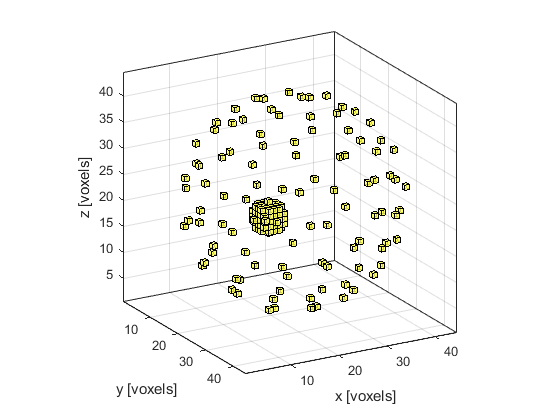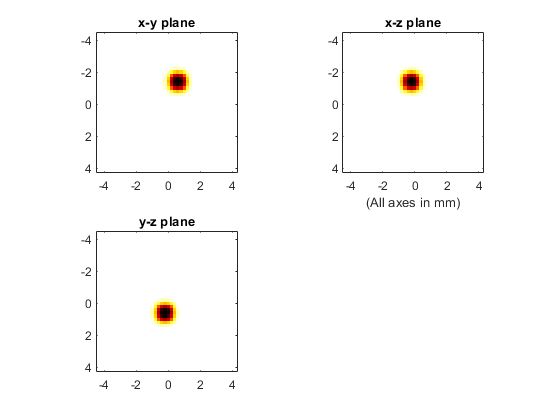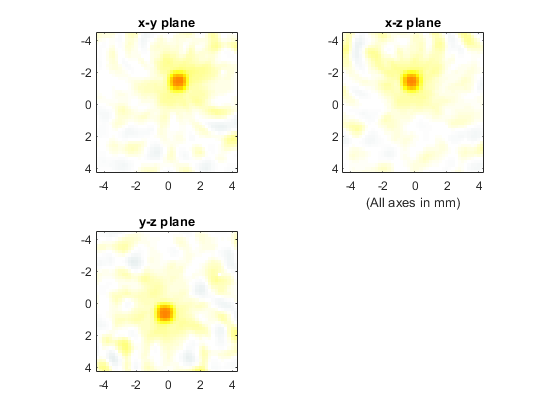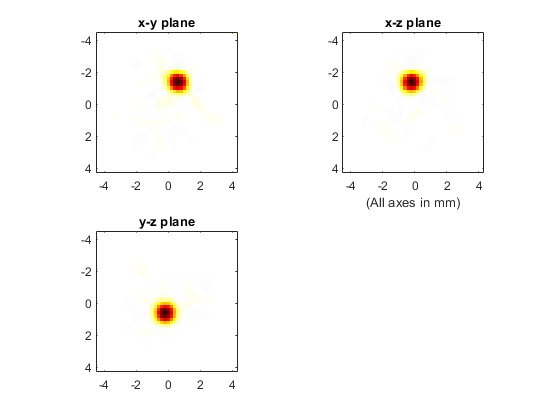# 3D Time Reversal Reconstruction For A Spherical Sensor Example

This example demonstrates the use of k-Wave for the time-reversal reconstruction of a three-dimensional photoacoustic wave-field recorded over a spherical sensor. The sensor data is simulated and then time-reversed using `kspaceFirstOrder3D`. It builds on the 2D Time Reversal Reconstruction For A Circular Sensor and 3D Time Reversal Reconstruction For A Planar Sensor examples.

## Simulating the sensor data

The sensor data is simulated using `kspaceFirstOrder3D` with an initial pressure distribution created using `makeBall`. A Cartesian sensor mask of 100 points evenly distributed around a sphere (using the Golden Section Spiral method) is created using `makeCartSphere`. A visualisation of the initial pressure and the sensor mask using `cart2grid` and `voxelPlot` is shown below.## Running the reconstruction

A plot of the initial pressure distribution and the reconstructed distribution using time-reversal are shown below (the three displayed image planes intersect at the centre of ball shown above). The plot scale for the reconstructed image has the plot limits reduced by a factor of 20.## Interpolating incomplete sensor data

The reconstruction can again be improved by interpolating the sensor data onto a continuous sensor surface. This is achieved using `interpCartData` and a binary sensor mask of a continuous sphere (created here using `makeSphere`) that is spatially equivalent to the Cartesian measurement grid. This function calculates the equivalent time-series at the sensor positions on the binary sensor mask from those on the Cartesian sensor mask via interpolation (nearest neighbour is used by default).

```% create a binary sensor mask of an equivalent continuous sphere

% interpolate data to remove the gaps and assign to time reversal data
```

Details of the interpolation are printed to the command line.

```Interpolating Cartesian sensor data...
interpolation mode: nearest
number of Cartesian sensor points: 100
number of binary sensor points: 4234
computation completed in 0.02934s
```

The reconstruction after interpolation is shown below. The plot limits are set to match those of the initial pressure plot shown above.